StatLect

# Impossible event

What is the definition of an impossible event in probability theory?

If you search on the web, you will find many sources that define it as an event whose probability is equal to zero. This definition is wrong.

Although the probability of an impossible event is zero, in many probabilistic models there are events that are not impossible but have zero probability.

The right definition might be a bit difficult to grasp: "the impossible event is the empty set".

What does this definition mean? In order to fully understand it, we need to recall how events are defined in probability theory.## Events

Remember that an event is a subset of the sample space, which is the set of all possible outcomes of a probabilistic experiment.

Example If the probabilistic experiment we are considering is the toss of a die, the sample space is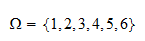The possible outcomes are the numbers fromto. An event is a subset of the sample space. So, for example,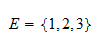andandare events.

## Definition of impossible event

In set theory, the empty setis the set that contains no elements. Given any set, the empty setis a subset of. In symbols: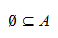So, given a sample space, the empty set is one of its subsets: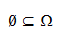It is an event and it is called the impossible event.

In other words, the impossible event is the event which does not contain any of the possible outcomes (remember that the possible outcomes are the elements of).

Let us see why this definition of impossible event is reasonable with an example.

Example Let us continue with the toss of a die experiment introduced in the previous example. Consider the event "the number that will appear face up is both greater than 4 and smaller than 2". This is obviously impossible because there is no number that satisfies these two conditions simultaneously. Let us see why the event is impossible also according to the formal definition. We are talking about two events. The event "greater than 4" is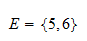and the event "smaller than 2" is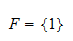The event "both greater than 4 and smaller than 2" is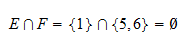Thus, it is impossible also according to the definition.

## The impossible event has zero probability

One of the basic properties of probability is that the empty set must have zero probability (see the lecture on Probability for a formal proof). Therefore, by definition, impossible events have zero probability.

## Not all zero-probability events are impossible

Although the impossible event has zero probability, not all zero-probability events are impossible. As a matter of fact, there are common probabilistic settings where the sample space is uncountable and each of the possible outcomes has zero probability. In other words, there are non-empty sets (events) that have zero probability.

## More details

If you are puzzled by the last statement (there are possible events with zero probability), you are advised to read the lecture on Zero-probability events.

If you want to know more about sample spaces and events, you can read the introductory lecture on Probability.

## Keep reading the glossary

Previous entry: IID sequence

Next entry: Information matrix